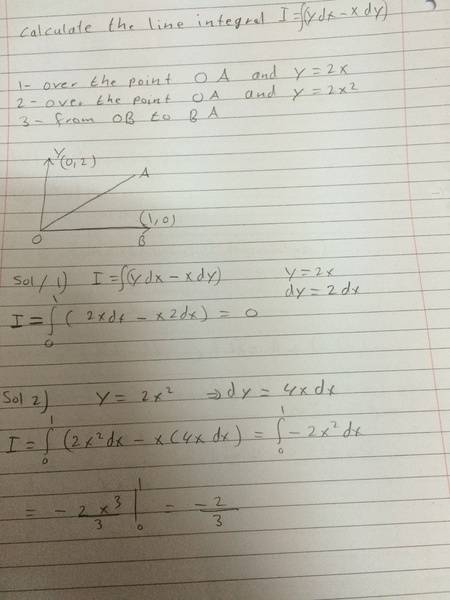# Calculate the line integral

• Pual Black
In summary, the student has difficulty solving the third homework problem and needs help understanding it. The student has shown that the line OB and the line BA are both possible paths from O to A, but they are not clear about what the third problem is asking.

## Homework Statement

i have this problem and need your help. I tried to solve the first 2 question but don't know ho to solve the third oneThe answer to your first part is wrong just fyi. Think about the area under the curve of that line. Does it look like it's zero?

As is the second part. You used the upper bound of the integral as the value you subtracted, it should be the thing you subtract from.

And I'm not sure what the third part is asking. Is it asking for the integral on the line OA and the line OB, in which case it'd be zero, or is there something you didn't write down. How is it exactly written in the problem?

Last edited:
Im not sure if i copied the problem right but i have another copy from my friend of the same problem

1- from OA ( straight line ) ##y=2x##
2- from OA ( arc ) ## y=2x^2 ##
3- the line OB
4- the line BA

What you have shown is difficult to read! It appears that you meant that O and A are points: O= (0, 0) and A= (1, 2). But if so, then "over the point OA" makes no sense. I think you mean "over the line OA".

If $y= 2x$, what is dy?

If $y= 2x^2$ what is dy?

I'm not clear on what "3- the line OB, 4- the line BA" is supposed to mean when initially the you had the single problem, "From OB to BA", which also makes no sense since you do not go "from" one line to another. I think that what you mean is that all these integrals are from O to A, the third problem being along the horizontal line from O to B, y= 0, and then along the vertical line from B to A, x= 1.
If y= 0, what is dy? If x= 1, what is dx?

Well if you think about it for parts 3 and 4, when you take an integral you're finding the area underneath the curve between it and the x-axis. So if there's no distance between the line and the x-axis, or it's perpendicular to the x-axis, there is no area underneath it, so it's zero.

Maybe i shall use greens theorem??
Will it make sense??